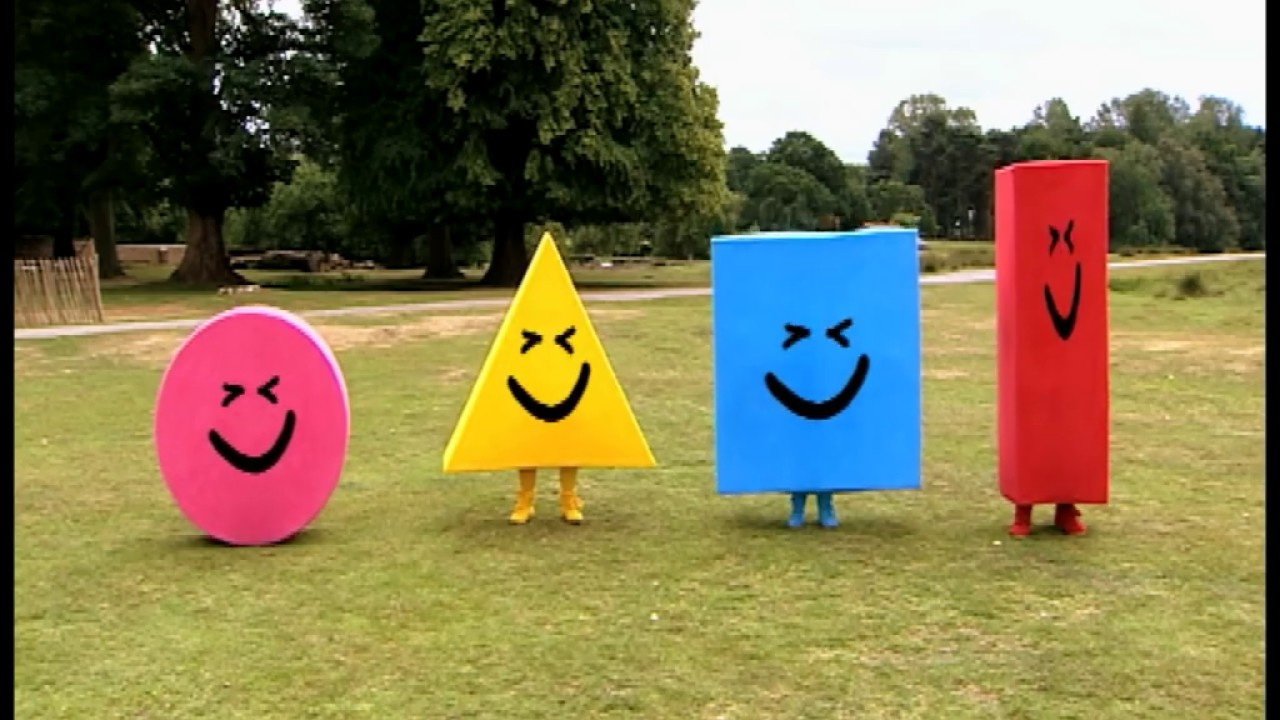# Year 8 Maths Chapter 3 - Measurement and Pythagoras’ theorem

You will want to refer to Year 7 Maths Chapter 10 for basic measurement skills. This section is more advanced, and omits area, length, perimeter, circumference, time, and other basic concepts.

## 2D Shapes

Special quadrilaterals include the parallelogram, rhombus, the kite and the trapezium. The formula for each 2D shape slightly varies.

Depending on your personal preference, you may use either the Textbook formula or the Standard formula.

Textbook formula is as follows:

``````Parallelogram - Base x Height (A = bh)
Rhombus and Kite - ½ x Diagonal x x Diagonal y (A = ½ xy)
Trapezium - ½ x Sum of parallel sides x Height (A = ½ h(a + b)
``````

However, you may prefer the Standard formula:

``````Parallelogram - A = bh where b is the base and h is the height
Rhombus and Kite - A = pq/2 where p and q each represent diagonals
Trapezium - A = ½ h(a + b) or A = ab/2 h where a and b are the sum of parallel sides and where h is the height
``````

The ratio of the area of a circle to the square of its radius is equal to π. Therefore, the area of a circle is known as `πr2` where r is the radius.

A half circle is known as a semi circle, and a quarter circle is known as a quadrant. Therefore, their respective area formulas are `½ πr2` and `¼ πr2`.

A sector’s area can be determined by calculating a fraction of the area of a circle with the same radius. Let ‘θ’ be the angle inside the sector.

``````Fraction = θ/360
Sector area = θ/360 x πr2
``````

A 2D composite shape is a shape that is composed of more than one basic shape (circle, square, triangle, etc.)And here is a composite shape, that can be split into multiple parts:The figure above, which we will call ‘N’, is made up of different size rectangles and squares.

The area of N, or any composite shape for the matter, can be found by adding or subtracting the areas of more basic shapes.

## 3D Shapes

A prism is a polyhedron (solid figure with multiple faces) with a constant cross-section.

The cross section is parallel to the two identical, congruent ends. The other sides are parallelograms or rectangles.

The volume of a prism, where V is the volume, A is the area of the cross section and h is the perpendicular height is:

``````V = Ah
``````

The volume of a cylinder is:

``````V = πr2h
``````

A net is a 2D representation of all surfaces unfolded. When folded, it forms the desired 3D outcome.

The surface area of a prism is the sum of the areas of all it’s faces. For example, a cube x with squares y, each with a side length of z would be calculated as:

``````A = 6z2
``````

## Capacity and volume

Volume is measured in cubic units.

• Cubic millimeters (mm3) [1000mm3 is equal to 1 milliliter]
• Cubic centimeter (cm3) [1cm3 is equal to 1 milliliter]
• Cubic meter (m3) [1m3 is equal to 1000 litres which is equal to 1 kilolitre]

Conversions for volume are as followed

``````     x10003           x1003            x103
km3 ----------> m3 ----------> cm3 -----------> mm3
/10003           / 1003           /103
``````

Capacity is the volume of liquids or gas that containers hold. In the metric system (the system that literally everyone except for the Americans use, oh wait, that’s celsius), there are some common units you should remember.

• Millilitre (mL)
• Litre (L)
• Kilolitre (kL)
• Megalitre (ML)

Some common conversions mentioned above might make more sense now.

• 1mL = 1cm3
• 1 L = 1000mL
• 1 kL = 1000L = 1m3

To find the volume of a rectangular prism, you would multiply the length by the breadth by the height.

``````V = length x breadth x height
``````

The volume of a cube (also a rectangular prism) is ‘V = s^3’ where s is one side length.

## Pythag

The hypotenuse is the longest side of a right angled triangle, opposite the right angle. Pythagoras’ theorem dictates that the square of the hypotenuse is the sum of the squares of the other two shorter sides.

Therefore, where a and b is the length of the side of the shorter sides, and c is the hypotenuse:

``````a² + b² = c²
``````

A Pythagorean triad (also known as a Pythagorean triple) is a set of three integers which satisfy Pythagoras’ theorem.

For example, 3-4-5 is a Pythagorean triad because `3² + 4² = 5²`, or `9 + 16 = 25`.

Surds are numbers that cannot be written as a fraction. Written as a decimal, the decimal numbers repeat, thus, surds are also irrational. Surds contain a √ sign when written in the simplest form.

Pythagoras’ theorem dictates that:

``````a² + b² = c²
therefore
√(a² + b²) = c
``````

where the brackets next to the √ sign indicate its constituents are under the square root.

However:

``````√(a² + b²) ≠ a + b
``````

Using your algebra skills, you can find the length of a shorter side using Pythagoras’ theorem.

For a triangle with sides a and 24, where 25 is the hypotenuse:

``````a² + b² = c²
a² + 24² = 25²
a² + 576 = 625
a² = 49 (Subtract 576 from both sides.)
∴ a = √49
= 7
``````

Copyright © 2017-2020 aidswidjaja and other contributors. CC BY-SA 4.0 Australia unless otherwise stated.